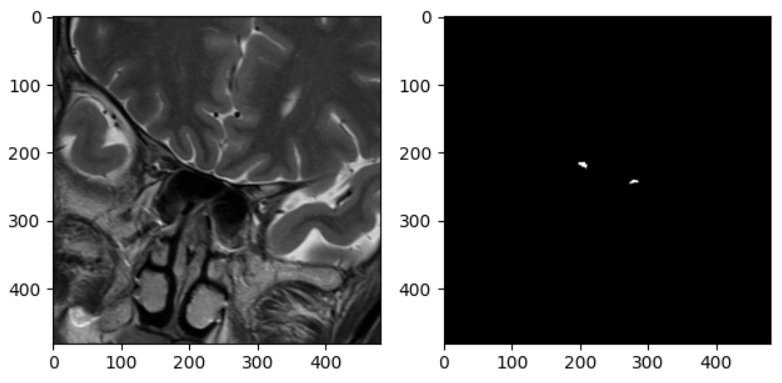# 数据增广时 input 和 target 保持相同变换

self.transform = transforms.Compose([
transforms.RandomHorizontalFlip(),
transforms.RandomRotation(90),
transforms.CenterCrop(480),
transforms.ToTensor(),
transforms.ToPILImage()
])

image = self.transform(image)
bulb = self.transform(bulb)

torchvision.transforms 内使用的是 python 原生 random 库（pytorch vision 1.5），在两次变换前指定相同的 random seed 即可。

seed = random.randint(0, 2 ** 32)
random.seed(seed)  # set the same seed to transform together
image = self.transform(image)
random.seed(seed)  # set the same seed to transform together
bulb = self.transform(bulb)

t = MRIDataset('/home/sunyf/dataset/ob/new', ['01', '02'])
import matplotlib.pyplot as plt
for i in range(20):
data = t[i]  # 测试时应该按照调用方式，只调用一次，否则两次seed不同
raw = data
fig = plt.figure()
plt.title(str(i))
plt.subplot(1, 2, 1), plt.imshow(raw, cmap='gray')
plt.show()raw = t[i]
mask = t[i]